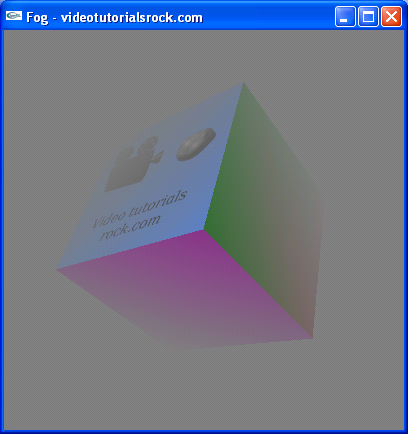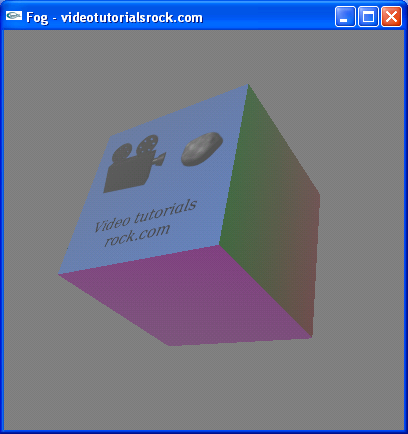# Lesson 15: Fog

The basic idea behind fog in OpenGL is that whenever we draw a pixel to the screen, we're going to average the color gray with the pixel. Pixels that are farther away from the camera have a heigher weighting for the gray color, while pixels closer to the camera have a lower weighting. In other words, pixels farther from the camera will be more gray.

## Adding Fog to the Spinning Cube Program

Let's add fog to the spinning cube program from this earlier lesson.

First of all, we'll add a call to glEnable(GL_FOG) to the initRendering function, to enable fog. We'll also call glClearColor(0.5f, 0.5f, 0.5f, 1) to change the background color to match the color of the fog. Then, we'll add some code to drawScene to set up the fog.

```void drawScene() {
//...
GLfloat fogColor[] = {0.5f, 0.5f, 0.5f, 1};
glFogfv(GL_FOG_COLOR, fogColor);
glFogi(GL_FOG_MODE, GL_LINEAR);
glFogf(GL_FOG_START, 10.0f);
glFogf(GL_FOG_END, 20.0f);
//...
}```

First of all, we'll let OpenGL know the color of the fog. The array fogColor stores the color that we will use for the fog; the first three elements are the RGB color of the fog, and the last element is 1. You could actually use fog that's red, green, or any kind of weird color, but we'll just use gray.

There's another important thing that OpenGL needs to know about the fog. It needs a function that takes the distance of a pixel from the camera and returns the weighting that will be assigned to the color gray. The weights range from 0, indicating no gray, to 1, indicating 100% gray. OpenGL allows us to use a few different functions. Below are the three functions that we'll try.First, we'll use the linear function. The linear function starts at 0 until we reach a certain distance, then linearly climbs to 1 at a second distance, then stays at 1. To indicate a linear function, we call glFogi(GL_FOG_MODE, GL_LINEAR). Then, we have to tell OpenGL the near and far distances that are needed for the function; in this case, we'll use distances of 10 and 20. We do this by calling glFogf(GL_FOG_START, 10.0f) and glFogf(GL_FOG_END, 20.0f). With this code, our program looks like this:We have a nice fog effect. Points on the cube that are closer to the camera are less gray, while points that are farther from the camera are more gray.

Now let's try an exponential function. We'll switch from GL_LINEAR to GL_EXP. Then, we comment out the calls to glFogf, since the near and far distances no longer have any meaning. They only matter for the linear function. In their place, we put a call to glFogf(GL_FOG_DENSITY, 0.05f), indicating a density parameter of 0.05. The function we've just set up is y = 1 - e-0.05x, where y is the weighting for the color gray, x is the distance from the camera, and 0.05 is the fog density. If we use this function, the program looks like this:It still kind of looks like there's fog, but it doesn't look as good as when we were using the linear function. There's less difference between the grayness of the different points on the cube. That's because most of the points on the cube are a distance of roughly 15 to 20 units from the camera. With an exponential function, this corresponds to a small range of weightings for the color gray. So, in this program, the linear function works better than an exponential function. In more complex scenes or in other programs, an exponential function might look better. For a given program, you need to try out each function and see which works best.

Now, we'll switch to an exponential-squared function. This is a function of the form y = 1 - e-(0.05x)2. To do this, we just have to change from GL_EXP to GL_EXP2. Like the exponential function, it uses the density parameter to indicate the constant in the function. If we do this, the program looks like this:This function also doesn't work as well as a linear function, but again, it may work better in other programs.

I've been saying that OpenGL computes the distance of each pixel from the camera, but I should mention that this may not be the case. OpenGL might just compute the distance from each vertex to the camera, and interpolate the fog effect across a face. I just thought I'd point that out.

Anyway, that's how fog works in OpenGL.

Next is "Part 4: Improving Speed and Appearance".